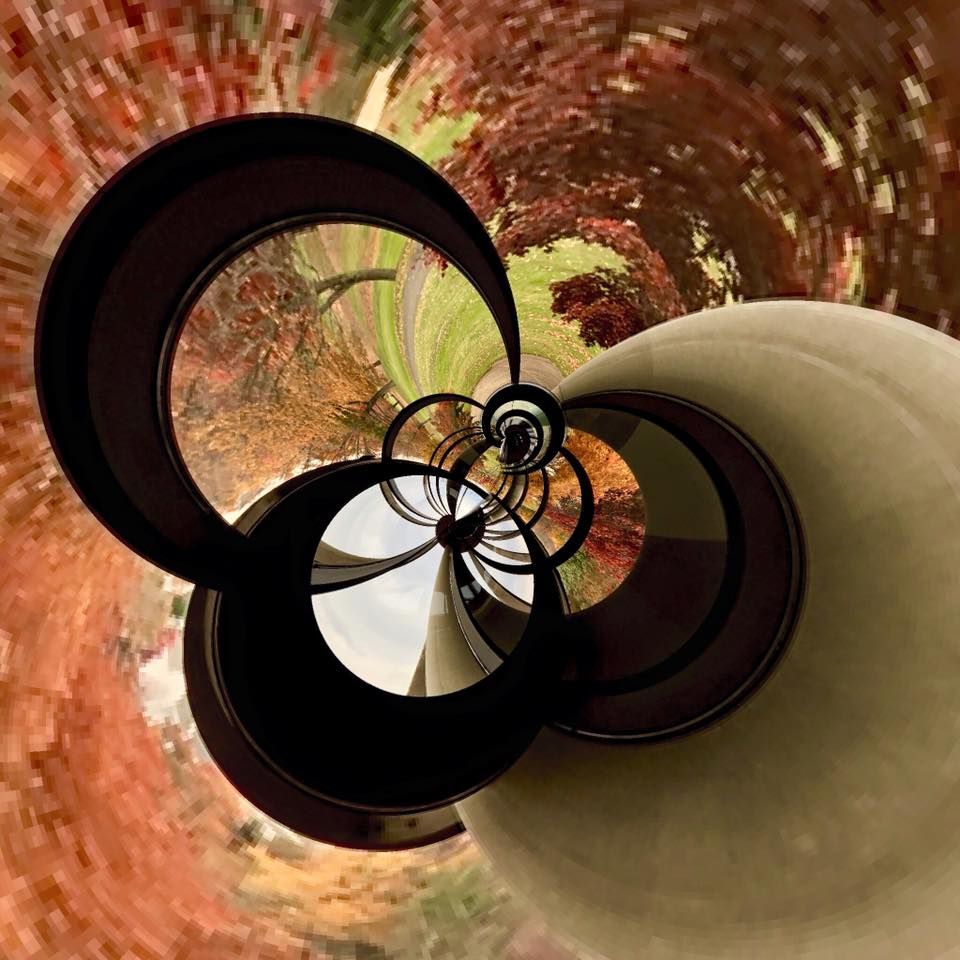## My Second (R) Shiny App: Sampling Distributions & CLTImage Credit: Doug Buckley of http://hyperactive.to

I was so excited about my initial foray into Shiny development using jennybc‘s amazing package that I stayed up half the night last night (again) working on my second Shiny app: a Shiny-fied version of the function I shared in March to do simulations illustrating sampling distributions and the Central Limit Theorem using many different source distributions. (Note that Cauchy doesn’t play by the rules!) Hope this info is useful to all new Shiny developers.

If the app doesn’t work for you, it’s possible that I’ve exhausted my purchased hours at http://shinyapps.io — no idea how much traffic this post might generate. So if that happens to you, please try getting Shiny to work locally, cutting and pasting the code below into server.R and ui.R files, and then launching the simulation from your R console.

Here are some important lessons I learned on my 2nd attempt at Shiny development:

• Creating a container (rv) for the server-side values that would change as a result of inputs from the UI was important. That container was then available to the portions of my Shiny code that prepared data for the UI, e.g. output\$plotSample.
• Because switch only takes arguments that are 1 character long, using radio buttons in the Shiny UI was really useful: I can map the label on each radio button to one character that will get passed into the data processing on the server side.
• I was able to modify the CSS for the page by adding a couple lines to mainPanel() in my UI.
• Although it was not mentally easy (for me) to convert from an R function to a Shiny app when initially presented with the problem, in retrospect, it was indeed straightforward. All I had to do was take the original function, split out the data processing from the presentation (par & hist commands), put the data processing code on the server side and the presentation code on the UI side, change the variable names on the server side so that they had the input\$ prefix, and make sure the variable names were consistent between server and UI.
• I originally tried writing one app.R file, but http://shinyapps.io did not seem to like that, so I put all the code that was not UI into the server side and tried deploying with server.R and ui.R, which worked. I don’t know what I did wrong.
• If you want to publish to http://shinyapps.io, the directory name that hosts your files must be at least 4 characters long or you will get a “validation error” when you attempt to deployApp().
```## Nicole's Second Shiny Demo App
## Used code from http://github.com/homerhanumat as a base
###########################################################
## ui
###########################################################

ui <- fluidPage(
titlePanel('Sampling Distributions and the Central Limit Theorem'),
sidebarPanel(
helpText('Choose your source distribution and number of items, n, in each
sample. 10000 replications will be run when you click "Sample Now".'),
href="http://www.r-bloggers.com/sampling-distributions-and-central-limit-theorem-in-r/", target="_blank")),
sliderInput(inputId="n","Sample Size n",value=30,min=5,max=100,step=2),
c("Exponential: Param1 = mean, Param2 = not used" = "E",
"Normal: Param1 = mean, Param2 = sd" = "N",
"Uniform: Param1 = min, Param2 = max" = "U",
"Poisson: Param1 = lambda, Param2 = not used" = "P",
"Cauchy: Param1 = location, Param2 = scale" = "C",
"Binomial: Param1 = size, Param2 = success prob" = "B",
"Gamma: Param1 = shape, Param2 = scale" = "G",
"Chi Square: Param1 = df, Param2 = ncp" = "X",
"Student t: Param1 = df, Param2 = not used" = "T")),
numericInput("param1","Parameter 1:",10),
numericInput("param2","Parameter 2:",2),
actionButton("takeSample","Sample Now")
), # end sidebarPanel
mainPanel(
# Use CSS to control the background color of the entire page
tags\$style("body {background-color: #9999aa; }")
),
plotOutput("plotSample")
) # end mainPanel
) # end UI

##############################################################
## server
##############################################################

library(shiny)
r <- 10000 # Number of replications... must be ->inf for sampling distribution!

palette(c("#E41A1C", "#377EB8", "#4DAF4A", "#984EA3",
"#FF7F00", "#FFFF33", "#A65628", "#F781BF", "#999999"))

server <- function(input, output) {
set.seed(as.numeric(Sys.time()))

# Create a reactive container for the data structures that the simulation
# will produce. The rv\$variables will be available to the sections of your
# server code that prepare output for the UI, e.g. output\$plotSample
rv <- reactiveValues(sample = NULL,
all.sums = NULL,
all.means = NULL,
all.vars = NULL)

# Note: We are giving observeEvent all the output connected to the UI actionButton.
# We can refer to input variables from our UI as input\$variablename
observeEvent(input\$takeSample,
{
my.samples <- switch(input\$src.dist,
"E" = matrix(rexp(input\$n*r,input\$param1),r),
"N" = matrix(rnorm(input\$n*r,input\$param1,input\$param2),r),
"U" = matrix(runif(input\$n*r,input\$param1,input\$param2),r),
"P" = matrix(rpois(input\$n*r,input\$param1),r),
"C" = matrix(rcauchy(input\$n*r,input\$param1,input\$param2),r),
"B" = matrix(rbinom(input\$n*r,input\$param1,input\$param2),r),
"G" = matrix(rgamma(input\$n*r,input\$param1,input\$param2),r),
"X" = matrix(rchisq(input\$n*r,input\$param1),r),
"T" = matrix(rt(input\$n*r,input\$param1),r))

# It was very important to make sure that rv contained numeric values for plotting:
rv\$sample <- as.numeric(my.samples[1,])
rv\$all.sums <- as.numeric(apply(my.samples,1,sum))
rv\$all.means <- as.numeric(apply(my.samples,1,mean))
rv\$all.vars <- as.numeric(apply(my.samples,1,var))
}
)

output\$plotSample <- renderPlot({
# Plot only when user input is submitted by clicking "Sample Now"
if (input\$takeSample) {
# Create a 2x2 plot area & leave a big space (5) at the top for title
par(mfrow=c(2,2), oma=c(0,0,5,0))
hist(rv\$sample, main="Distribution of One Sample",
ylab="Frequency",col=1)
hist(rv\$all.sums, main="Sampling Distribution of the Sum",
ylab="Frequency",col=2)
hist(rv\$all.means, main="Sampling Distribution of the Mean",
ylab="Frequency",col=3)
hist(rv\$all.vars, main="Sampling Distribution of the Variance",
ylab="Frequency",col=4)
mtext("Simulation Results", outer=TRUE, cex=3)
}
}, height=660, width=900) # end plotSample

} # end server```

•“Because switch only takes arguments that are 1 character long, using radio buttons in the Shiny UI was really useful”. switch() takes an expression evaluating to a number or a character *string*, which means can be much longer than a single character. This doesn’t mean that you should (or shouldn’t) use radio buttons, but you don;t have to restrict yourself because of switch.••